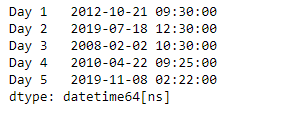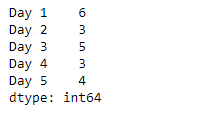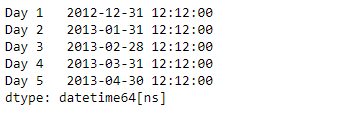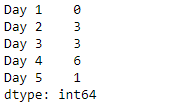# Python | Pandas Series.dt.weekday

• Last Updated : 20 Mar, 2019

`Series.dt` can be used to access the values of the series as datetimelike and return several properties. Pandas` Series.dt.weekday` attribute return the day of the week. It is assumed the week starts on Monday, which is denoted by 0 and ends on Sunday which is denoted by 6.

Syntax: Series.dt.weekday

Attention geek! Strengthen your foundations with the Python Programming Foundation Course and learn the basics.

To begin with, your interview preparations Enhance your Data Structures concepts with the Python DS Course. And to begin with your Machine Learning Journey, join the Machine Learning - Basic Level Course

Parameter : None

Returns : numpy array

Example #1: Use `Series.dt.weekday` attribute to return the day of week for the given datetime in the underlying data of the given Series object.

 `# importing pandas as pd``import` `pandas as pd`` ` `# Creating the Series``sr ``=` `pd.Series([``'2012-10-21 09:30'``, ``'2019-7-18 12:30'``, ``'2008-02-2 10:30'``,``               ``'2010-4-22 09:25'``, ``'2019-11-8 02:22'``])`` ` `# Creating the index``idx ``=` `[``'Day 1'``, ``'Day 2'``, ``'Day 3'``, ``'Day 4'``, ``'Day 5'``]`` ` `# set the index``sr.index ``=` `idx`` ` `# Convert the underlying data to datetime ``sr ``=` `pd.to_datetime(sr)`` ` `# Print the series``print``(sr)`

Output :Now we will use `Series.dt.weekday` attribute to return the day of week for the given datetime in the underlying data of the given Series object.

 `# return the day of week``result ``=` `sr.dt.weekday`` ` `# print the result``print``(result)`

Output :As we can see in the output, the `Series.dt.weekday` attribute has successfully accessed and returned the day of week in the underlying data of the given series object.

Example #2 : Use `Series.dt.weekday` attribute to return the day of week for the given datetime in the underlying data of the given Series object.

 `# importing pandas as pd``import` `pandas as pd`` ` `# Creating the Series``sr ``=` `pd.Series(pd.date_range(``'2012-12-12 12:12'``, periods ``=` `5``, freq ``=` `'M'``))`` ` `# Creating the index``idx ``=` `[``'Day 1'``, ``'Day 2'``, ``'Day 3'``, ``'Day 4'``, ``'Day 5'``]`` ` `# set the index``sr.index ``=` `idx`` ` `# Print the series``print``(sr)`

Output :Now we will use `Series.dt.weekday` attribute to return the day of week for the given datetime in the underlying data of the given Series object.

 `# return the day of week``result ``=` `sr.dt.weekday`` ` `# print the result``print``(result)`

Output :As we can see in the output, the `Series.dt.weekday` attribute has successfully accessed and returned the day of week in the underlying data of the given series object.

My Personal Notes arrow_drop_up